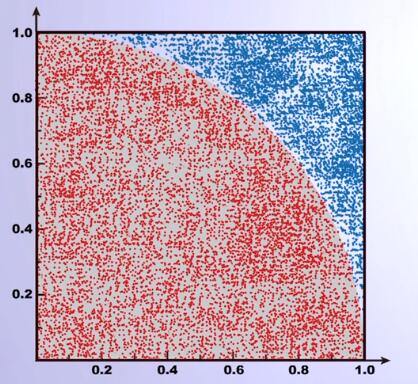• 单峰分布数学期望值计算方法的探究，孔建新，孔璐，单峰分布密度函数建立确定其数学期望值是分布峰值点上的取值：众数。其取值的计算方法与传统给出的众数计算方法存在不一致的问题
• 国际公认的π值计算采用蒙特卡洛方法。 蒙特卡洛方法 蒙特卡洛(Monte Carlo)方法，又称随机抽样或统计试验方法。当所求解的问题是某种事件出现的概率，或某随机变量的期望值时，可以通过某种“试验”方法求解。 ...
圆周率π是一个无理数，没有任何一个精确公式能够计算π值，π的计算只能采用近似算法。

国际公认的π值计算采用蒙特卡洛方法。

蒙特卡洛方法

蒙特卡洛(Monte Carlo)方法，又称随机抽样或统计试验方法。当所求解的问题是某种事件出现的概率，或某随机变量的期望值时，可以通过某种“试验”方法求解。

简单说，蒙特卡洛是利用随机试验求解问题的方法。

π值的计算

构造一个单位正方形和一个单位圆的1/4，往整个区域内随机投入点，根据点到原点的距离判断点是落在1/4的圆内还是在圆外，从而根据落在两个不同区域的点的数目，求出两个区域的比值。如此一来，就可以求出1/4单位圆的面积，从而求出圆周率π。下面是用Python的实现：

# pi.py
from random import random
from math import sqrt
from time import clock
DARTS = 1200
hits = 0
clock()
for i in range(1, DARTS):
x, y = random(), random();
dist = sqrt(x**2 + y**2)
if dist <= 1.0:
hits = hits + 1
pi = 4 * (hits/DARTS)
print('Pi的值是 %s' % pi)
print('程序的运行时间是 %-5.5ss' % clock())

代码中用到了random和math库的random()函数和sqrt()函数，为了统计时间，还用到了time库的clock()函数。

投入的点越多，计算值越精确。

结语

蒙特卡洛方法提供了一个利用计算机中随机数和随机试验来解决现实中无法用公式求解问题的思路，广泛应用在金融工程学、宏观经济学、计算物理学等领域。
展开全文Python
• 这道题网络上的解法似乎存在问题。因此我在这里写出自己的方法
这是《算法导论》第三版的题目5.2-3：利用指示器随机变量计算掷n次骰子总和的期望值。解：定义事件A为一次投掷结果小于等于1，B为一次投掷结果小于等于2，C为一次投掷结果小于等于3，D为一次投掷结果小于等于4，E为一次投掷结果小于等于5，F为一次投掷结果小于等于6。定义指示器随机变量I{·}为：                            I{x}=1   如果x发生，否则为0一次投掷结果的期望E(X1)=E(I(A)+I(B)+I(C)+I(D)+I(E)+I(F))=1/6+2/6+3/6+4/6+5/6+6/6=3.5那么n次投掷结果的期望E(X1+X2+...+Xn)=3.5n
展开全文指示器随机变量
• 三刺激值计算是求取各种色度量的基础。首先回顾了国际照明委员会三刺激值的基本定义及其数值计算方法;其次简单描述和分析了相关工业界常用的三种ASTM加权表及Venable最优加权表;最后,基于Venable的工作,从减小光谱...
• 在matlab中，我们使用qqplot（）来生成QQplot图，但是这并不意味这我们能够很容易的计算并得到GIF，我们生成QQplot以后才能从头计算GIF。 第一步就是，我们首先得到正态分布的期望顺序统计量；正态分
GIF值通常用在GWA过程中，用来控制该过程质量；鉴别出那些低质量的基因标记；例如在统计学中，GIF值大于1.0表示结果有低质量数据的出现；
那么我们如何计算gwa中的GIF呢；在matlab中，我们使用qqplot（）来生成QQplot图，但是这并不意味这我们能够很容易的计算并得到GIF值，我们生成QQplot以后才能从头计算GIF。
第一步就是，我们首先得到正态分布的期望顺序统计量；正态分布的第i次顺序统计量服从参数（i，n-i+1）的贝塔分布，期望值是：$E \left\{p_{(i)} \right\} = i / (n+1) \; \; \; \; (i=1,\ldots,n)$
这个是比较容易计算的。下面是个简单的MATLAB程序来显示GWA分析的QQplot并计算GIF：

function gif=compute_gif(pvals)

% number of p-vals
n=length(pvals);

% expected order statistics
es=(1:n)' ./ (n+1);

% x-axis
x = -log10(es);
y = -log10(sort(pvals(:)));

% compute GIF
gif =(x'*y)/(x'*x);

% QQ-plot
figure;
hold;
grid;
maxh=ceil(log10(n));
xlim([0 maxh]);
ylim([0 maxh]);
plot(x,y,'bx');
plot(x,x,'r-');
xlabel('-log10(Expected Order Statistics)');
ylabel('-log10(Observed Order Statistics)');
title('QQ Plot');

% done
return;
函数compute_gif() 只有一个参数，这是p值的list，对p值进行排序并使用该排序生成QQplot计算GIF。

展开全文statistics matlab plot function list
• 对非经典分布的随机变量，当然可以按博文《自定义离散型分布》中介绍的方法，自定义rv_discrete（离散型）或rv_continuos（连续型）的子类（详见博文《自定义连续型分布》），然后调用其expect函数计算数学期望。...
对非经典分布的随机变量，当然可以按博文《自定义离散型分布》中介绍的方法，自定义rv_discrete（离散型）或rv_continuos（连续型）的子类（详见博文《自定义连续型分布》），然后调用其expect函数计算数学期望。如果仅为计算随机变量的数学期望，也可以对表示分布律的数组（离散型）或密度函数（连续型），按定义直接计算。
注意到，对于取有限多个值离散型随机变量
$X\text{\~{}}\begin{pmatrix}x_1&x_2&\cdots&x_n\\p_1&p_2&\cdots&p_n\end{pmatrix}$
其函数$Y=g(X)$的期望$E(g(X))=\sum\limits_{i=1}^ng(x_i)p_i$是数组$[g(x_1),g(x_2),\cdots,g(x_n)]$与数组$[p_1,p_2,\cdots,p_n]$按元素相乘后所得数组$[g(x_1)p_1,g(x_2)p_2,\cdots,g(x_n)p_n]$元素的和。而联合分布律为的2-维离散型随机向量$(X,Y)$，其函数$g(X,Y)$的数学期望$E(g(X,Y))=\sum\limits_{i=1}^m\sum\limits_{j=1}^ng(x_i,y_j)p_{ij}$是2-维数组$\begin{pmatrix}g(x_1,y_1)&g(x_1,y_2)&\cdots&g(x_1,y_n)\\g(x_2,y_1)&g(x_2,y_2)&\cdots&g(x_2,y_n)\\\vdots&\vdots&\cdots&\vdots\\g(x_m,y_1)&g(x_m,y_2)&\cdots&g(x_m,y_n)\end{pmatrix}$和$\begin{pmatrix}p_{11}&p_{12}&\cdots&p_{1n}\\p_{21}&p_{22}&\cdots&p_{2n}\\\vdots&\vdots&\cdots&\vdots\\p_{m1}&p_{m2}&\cdots&p_{mn}\end{pmatrix}$按元素相乘所得2-维数组$\begin{pmatrix}g(x_1,y_1)p_{11}&g(x_1,y_2)p_{12}&\cdots&g(x_1,y_n)p_{1n}\\g(x_2,y_1)p_{21}&g(x_2,y_2)p_{22}&\cdots&g(x_2,y_n)p_{2n}\\\vdots&\vdots&\cdots&\vdots\\g(x_m,y_1)p_{m1}&g(x_m,y_2)p_{m2}&\cdots&g(x_m,y_n)p_{mn}\end{pmatrix}$的元素之和。
对比两者，仅仅是参加计算的数组结构（前者是1-维数组，后者是2-维数组）与函数自变量个数（前者是一元函数，后者是二元函数）不同，计算方法是一样的。我们可以写成一个统一的计算数学期望的函数
def expect(P, Xv=None, Yv=None, func=lambda x, y: x):
stru=P.shape                                #获取P的结构
arrayType=type(np.array([]))                #数组类型
if (len(stru)>1) and (type(Xv)==arrayType): #2维向量且需计算X
Xv=Xv.reshape(Xv.size,1)
if type(Yv)==arrayType:                     #2维向量且需计算Y
Yv=Yv.reshape(1, Yv.size)
mean=(func(Xv,Yv)*P).sum()                  #计算期望
return mean

函数expect的4个参数中P表示分布律中的概率序列。Xv和Yv分别表示随机变量$X$和$Y$的取值序列，缺省为None。func表示函数关系$Z=g(X,Y)$，缺省值为函数$g(X,Y)=X$。之所以表示成二元函数，是因为我们用统一的形式，计算随机变量的函数或随机向量的函数。第2行读取表示概率序列的数组P的结构，P.shape是一个元组，其长度大于1表示P是一个矩阵。第3行获取numpy的数组类型，记为arrayType，若参数Xv或Yv传递的是数组，则其类型type(Xv)（或type(Yv)）就与arrayType一致。第4~5行的if语句对2-维随机向量（len(stru)>1）且需计算$X$期望（type(Xv)==arrayType）的情形，将Xv设置成$m\times1$的列向量，以保证矩阵按元素计算的正确性。出于同样的目的，第6~7行的if语句对需要计算$Y$的期望（此时，P一定是2-维数组），将Yv设置成$1\times n$的行向量。第8行将数组func(Xv,Yv)*P元素之和(func(Xv,Yv)*P).sum()记为返回值mean。第9行将计算结果返回。
例1 有3只球，4个盒子，盒子的编号为1、2、3。将球逐个独立地，随机地放入4个盒子中去。以$X$表示其中至少有一只球的盒子的最小号码（例如$X=3$表示第1号，第2号盒子是空的，第3号盒子至少有一只球），计算$E(X)$。
解： 显然，$X$的取值为$\{1, 2, 3, 4\}$。设$A_i$表示$i$号盒是空的（$i=1, 2, 3, 4$）。每个球放入1号盒的概率为$1/4$，没有放入1号盒的概率为$3/4$。
$P(X=1)=P(\overline{A}_1)=1-P(A_1)=1-\left(\frac{3}{4}\right)^3=\frac{37}{64}$
$P(X=2)=P(A_1\overline{A}_2)=P(A_1)P(\overline{A}_2|A_1)\\ =P(A_1)(1-P(A_2|A_1))=\left(\frac{3}{4}\right)^3\left[1-\left(\frac{2}{3}\right)^3\right]\\ =\frac{27}{64}\cdot\frac{19}{27}=\frac{19}{64}$
$P(X=3)=P(A_1A_2\overline{A}_3)=P(A_1)P(A_2|A_1)P(\overline{A}_3|A_1A_2)\\ =\left(\frac{3}{4}\right)^3\left(\frac{2}{3}\right)^3\left[1-\left(\frac{1}{2}\right)^3\right]=\frac{7}{64}$
$P(X=4)=P(A_1A_2A_3\overline{A}_4)=P(A_1)P(A_2|A_1)P(A_3|A_1A_2)P(\overline{A}_4|A_1A_2A_3)\\ =\left(\frac{3}{4}\right)^3\left(\frac{2}{3}\right)^3\left(\frac{1}{2}\right)^3=\frac{1}{64}$
即$X$~$\begin{pmatrix}1&2&3&4\\\frac{37}{64}&\frac{19}{64}&\frac{7}{64}&\frac{1}{64}\end{pmatrix}$，$E(X)=1\cdot\frac{37}{64}+2\cdot\frac{19}{64}+3\cdot\frac{7}{64}+4\cdot\frac{1}{64}=\frac{25}{16}$。下列代码调用上列程序定义的expect函数验算$E(X)$。
import numpy as np                              #导入numpy
from sympy import Rational                      #导入Rational
X=np.array([1, 2, 3, 4])                        #设置X的取值
P=np.array([Rational(37,64), Rational(19, 64),  #设置取值对应的概率
Rational(7,64), Rational(1,64)])
mean=expect(P, X)                               #计算数学期望
print('E(X)=%s'%mean)

注意第6行调用上列程序定义的expect函数，仅传递参数P和X，其余参数均缺省，计算随机变量$X$的数学期望$E(X)$。运行程序，输出
E(X)=25/16

对于二维离散型随机向量$(X,Y)$函数$g(X,Y)$的数学期望的计算，调用expect函数时，需正确传递参数。
例2 设$(X, Y)$的联合分布律为计算$E(X)$，$E(Y)$和$E(X^3Y^2)$。
解： 为计算$E(X)$和$E(Y)$，先计算$X$，$Y$的边缘分布。不难解得$X$~$\begin{pmatrix}0&1\\\frac{5}{8}&\frac{3}{8}\end{pmatrix}$，$Y$~$\begin{pmatrix}1&2&3\\\frac{3}{8}&\frac{2}{8}&\frac{3}{8}\end{pmatrix}$。所以
$E(X)=0\times\frac{5}{8}+1\times\frac{3}{8}=\frac{3}{8}$,
$E(Y)=1\times\frac{3}{8}+2\times\frac{2}{8}+3\times\frac{3}{8}=2$。
为计算$E(X^3Y^2)$，可以运用先计算$Z=X^3Y^2$的分布律，然后计算$E(Z)$。根据$(X,Y)$的联合分布律，不难算得$Z$~$\begin{pmatrix}0&1&4&9\\\frac{5}{4}&\frac{1}{8}&\frac{1}{8}&\frac{1}{8}\end{pmatrix}$。于是$E(X^3Y^2)=E(Z)=0\times\frac{5}{4}+1\times\frac{1}{8}+4\times\frac{1}{8}+9\times\frac{1}{8}=\frac{7}{4}$。下列代码验算本例计算结果。
import numpy as np                      #导入numpy
from sympy import Rational as R         #导入Rational
X=np.array([0, 1])                      #设置X取值
Y=np.array([1, 2, 3])                   #设置Y取值
Pxy=np.array([[R(1,4), R(1,8), R(1,4)], #设置分布律中概率矩阵
[R(1,8), R(1,8), R(1,8)]])
meanx=expect(Pxy, X)                    #计算E(X)
g=lambda x, y: y                        #设置函数g(X,Y)=Y
meany=expect(Pxy, Yv=Y, Py)             #计算E(Y)
g=lambda x, y: (x**3)*(y**2)            #设置函数g(X, Y)=X^3Y^2
mean=expect(Pxy, X, Y, g)               #计算E(X^3Y^2)
print('E(X)=%.4f'%meanx)
print('E(Y)=%.4f'%meany)
print('E(X^3Y^2)=%.4f'%mean)

程序中第3~6行设置$(X,Y)$的联合分布律。第7行调用函数expect，传递参数Pxy和X计算$X$的边缘分布的期望$E(X)$，记为meanx。为计算$Y$的边缘分布期望$E(Y)$，第8行设置函数$g(X,Y)=Y$，第9行调用函数expect传递参数Pxy，Y和g，计算结果记为meany。第10行定义函数$g(x,y)=x^3y^2$，第11行调用函数expect传递参数Pxy，X，Y和g计算$E(X^3Y^2)$，记为mean。运行程序，输出
E(X)=3/8
E(Y)=2
E(X^3Y^2)=7/4

写博不易，敬请支持：
如果阅读本文于您有所获，敬请点赞、评论、收藏，谢谢大家的支持！


展开全文• 该方法根据服务消费者期望值选择QoS 因子，利用QoS 权重值计算Web 服务的质量，在此基础上给出一个基于QoS 计算的 Web 服务组合模型，并通过实例计算，证明利用该QoS 计算方法可以得到更高质量的增值服务。
• 参数估计的计算方法极大后验(MAP)及拉普拉斯逼近基于马尔可夫链的蒙特卡洛参数推断(MCMC)期望极大化(EM) （参数估计所有内容） 极大后验(MAP)及拉普拉斯逼近 极大后验估计： MAP是通过确定后验分布的极大得到的，...
• 这导致条件期望值的更简单计算。 算法（但不是估计条件期望值）也在、、中描述。 一个应用程序在中提供了将复杂的Ginzburg-Landau方程拟合到二元流体数据的方法。 用法： 程序 ace_main.m 包含数据的...matlab
• 在本文中，我们使用光锥晶格正则化方法计算了在质子激发模型中纯费米子态之间复合算子ΨΨ$$\ overline {\ varPsi} \ varPsi$$的有限体积期望值。 在圆锥形正则化图片中，此期望值与位于晶格相邻位置的晶格自旋...
• 基于该模型推导直流网络潮流计算方法。采用直流电压下垂控制的换流器其直流输送功率会与参考偏离，为了提高直流系统输送能力，提出了根据期望输送功率对直流功率参考进行修正的方法。最后通过PSCAD／EMTDC时域...
• 定义了多值中智集、多值中智数及其期望值以及多值中智数的Hamming 距离, 并给出了其比较方法. 针对准则值为多值中智数的多准则决策问题, 将传统的交互式多准则决策(TODIM) 方法扩展到多值中智环境中, 提出一种基于多...
• 摘要： 1. 数学期望值, 平均值, mean http://zh.wikipedia.org/wiki/%E6%9C%9F%E6%9C%9B%E5%80%BC 表示: E[X], 即X变量的平均值. 也用miu表示 : μ=E[X] ...1. 数学期望值, 平均值, mean http://zh.wikipedia.
• 方法首先通过计算指标相对于指标期望的损益, 将决策矩阵转化为关于指标期望的损益矩阵; 然后运用随机占优准则, 通过判断针对每个指标两两方案之间的随机占优关系, 进而构建两两方案的随机占优关系矩阵, 并...
• 计算公式=工作质量加和的平均分*30%+工作量加和的平均分*20%+工作速度加和的平均分*20%+合作性加和的平均分*15%+工作态度加和的平均分*15%=总分 ...仅考虑工作的品质，与期望值比较，工作过程、结果的符合程度 ...
• 方法利用期望效用函数构建各准则的效用矩阵; 利用后悔-欣喜函数构建各准则相对于准则期望水平的后悔-欣喜矩阵; 在此基础上, 依据后悔理论构建各准则相对于准则期望水平的感知价值矩阵; 进一步, 利用...
• 然后, 在此基础上, 以匹配主体对各属性的期望作为参考点, 获得一方匹配主体对另一方相对于参考点的损益决策矩阵, 依据前景理论分别计算出匹配主体之间满意度的综合前景, 并进一步构建双目标优化模型; 最后, 通过...
• 方法首先通过计算指标相对于指标期望的损益，将决策矩阵转化为关于指标期望的损益矩阵；然后运用随机占优准则，通过判断针对每个指标两两方案之间的随机占优关系，进而构建两两方案的随机占优关系矩阵，并使用...
• 归纳出频度统计法、频度估算法、频度未知数法、列举频度归纳法、频度期望值法、扩展递归迭代法、上下限猜测法等几种根据算法特性求解时间复杂度的方法.这些方法涵盖了大多类算法,无论是在软件设计,还是在教学实践中,...算法效率分析
• Python计算获得多资产投资组合的风险度量。 关键概念 随着价格的变动，投资经理所...请注意，期望收益不是投资者认为他们将获得的收益，而是反映了所有经济情况下所有可能结果的平均。 风险价值（VaR）告诉.Python 风险价值 VaR
• 当所求解问题是某种随机事件出现的概率，或者是某个随机变量的期望值时，通过某种“实验”的方法，以这种事件出现的频率估计这一随机事件的概率，或者得到这个随机变量的某些数字特征，并将其作为问题的解。...
• 一个容斥的方法，(i^n-(i-1)^n)/m^n，就是最大为i的概率，因为在1-i里选的所有方案数减去1-(i-1)中选数字的方案数，就是1-i里选而且必包含i的方案总数。 #include&lt;bits/stdc++.h&gt; #define maxl ...
• 治疗效果的分布将普遍关注的平均治疗效果扩展到结果变量的尾部，分位数治疗效果表示在存在混杂机制的情况下计算这些效果的主要技术。 潜在的分位数回归基于 L1{损失函数，我们提出了期望治疗效果技术，该技术依赖于...
• bioRxiv 2020）提出的基于监督的基于多图集的基于期望的标签（ELV）方法的医学图像软分割的公共Matlab实现。 这种方法考虑了所有可能的图集到图像转换的可能性，并计算了ELV，从而绕过了可变形配准并避免了相关的...matlab
• 此外，当执行N * 2 ^ n查询非常昂贵时，第二种方法为相对较小的N提供了更可靠的输出，因为可以通过选择多个随机tweakey来更多地考虑平均的影响。 例子1 在此示例中，使用这两种方法，我们评估了6至18轮SKINNY-...C
• 将训练样本集作为每一个进化个体解码后的网络输入及输出，并将样本的期望输出同网络实际输出的平均误差平方和作为进化个体的适应度函数，将不同隐层节点数构成的进化个体的最优作为设计问题的高斯径向基网络结构．...
• 国际公认的PI值计算采用蒙特卡洛方法。 蒙特卡洛(Monte Carlo)方法,又称随机抽样或统计试验方法。 　当所求解问题是某种事件出现的概率,或某随机变量期望值时,可以通过某种“试验”的方法求解。 　即：...
• 在分析了风速离散性与连续性特点基础上，通过引入连续型风速的weibull概率分布和数学期望表达式，分别给出离散型和连续型风速数学期望计算方法。为求解风速的Weibull数学期望，首先提出用梯度法求出weibull函数的......